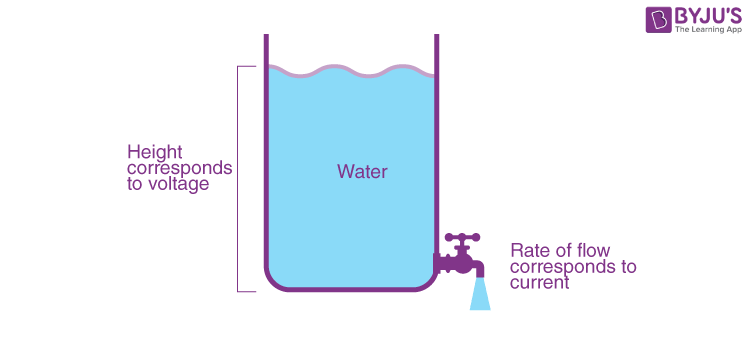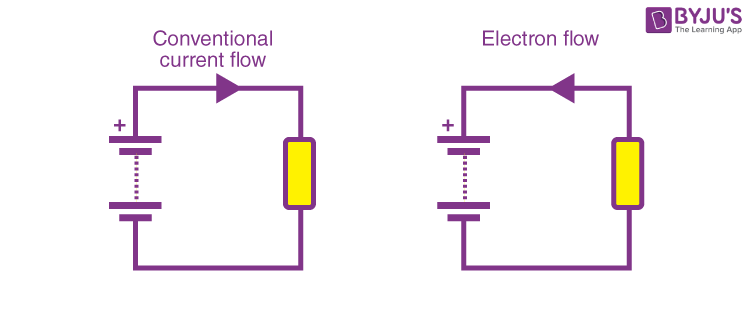# Electrical Current

The electrical current in the basic term is the flow of electric charge. The common electric charge carriers are electrons, but they can also be ions as in the case of chemical batteries. To understand how electrical current works let us look at the flow of water as an analogy.Consider a tall container fitted with a tap at the bottom. When you fill the container with water and turn on the tap, look at the flow of water. The amount of water flowing through the tap depends on many factors. These factors are as follows:

1. It depends on how much the tap is opened. A fully open tap allows more water flow as it offers less resistance, whereas a half-open tap lets less water flow as it has resistance to the flow of water, as the opening is small.
2. It also depends on the volume of water present in the container as this applies pressure. So if the volume is more, the pressure amount will increase simultaneously. Hence the flow of water will increase. Consequently, as the volume of water reduces, even the flow of water will reduce.

### What Consist of Electrical Current?

The SI unit of electric current is Ampere (A), 1 ampere is the amount of current when there is a flow of 1 coulomb of charge passing through a cross-section in 1 second.

Metals consisting of electric wires and other electronic equipment like copper, gold, and aluminium have many free electrons, which are responsible for the majority of the current flow. But in some semiconductors, depending on the type of dopant used, there can be positive charge carriers that flow in the opposite direction of that of electrons. In other cases, there can be both positive ions and negative electrons present at the same time as in a chemical battery. Therefore there will be confusion in the direction of flow of current. Hence a convention for the flow of current was introduced, which states that the direction of flow of current is in the positive direction, i.e. opposite to that of the flow of electrons.### Types of Electrical Current

There are two basic types of electrical currents depending on how they flow.

### Direct current (DC)

In this type, the current flows in only one direction, the advantage of this type of current is that it is very easy to store because almost all types of batteries use direct current, most electronics use direct current, computers, telephones, and satellites all work on DC.

### Alternating current(AC)

In this type, the current changes its direction, and the graph of AC looks like a sine wave, which means the current flows to and fro, our household current changes directions 50 times a second; hence you can see 50Hz written on most of our electrical equipment.

## The below videos help to revise the chapter Electricity Class 10## Frequently Asked Questions – FAQs

Q1

### What is electric current?

The flow of electric charge is referred to as electric current.
Q2

### What is the SI unit of electric current?

The SI unit of electric current is Ampere (A).
Q3

### Define 1 ampere.

1 ampere is the amount of current when there is a flow of 1 coulomb of charge passing through a cross-section in 1 second.
Q4

### What are the types of electrical current?

Types of Electrical Current are:
• Direct Current (DC)
• Alternating current (AC)
• Q5

### Telephones operate on which type of current?

Telephones operate on direct current.

#### Magnetic Effects of Electric Current#### NCERT Explanation Electricity Class 10Stay tuned with “BYJU’S – The learning app” for more such interesting information with engaging videos!

Test Your Knowledge On Electrical Current!